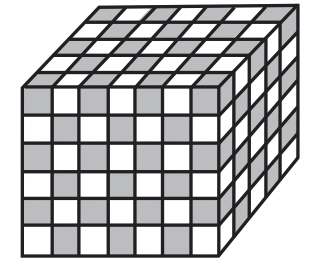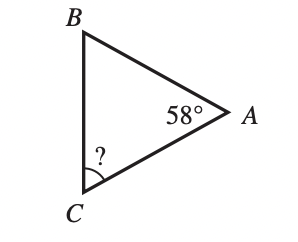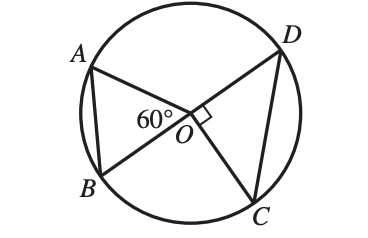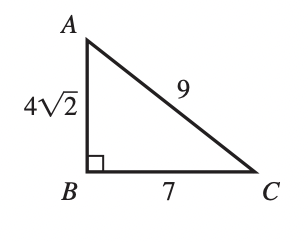# ACT Mathematics: Basic concept of Quadrilaterals Quiz

.This is an MCQ-based quiz on the topic of ACT Mathematics: Basic concept of Quadrilaterals Quiz.

This set of MCQs helps you brush up on the important mathematics topic and prepare you to dive into skill practice and expand your knowledge to tackle the question and answer it carefully.

Start Quiz

One side of square ABCD has a length of 12 meters. A certain rectangle whose area is equal to the area of ABCD has a width of 8 meters. What is the length, in meters, of the rectangle?

12

16

18

20

The solid rectangular prism shown below was built by alternating congruent black cubes and white cubes such that 2 cubes of the same color have at most 1 edge touching. What is the total number of white cubes that were used to build the prism?45

102

105

140

The solid rectangular prism shown below was built by alternating congruent black cubes and white cubes such that 2 cubes of the same color have at most 1 edge touching. What is the total number of white cubes that were used to build the prism?45

102

105

140

In ABC shown below, the measure of ∠A is 58°, and AB ≅ AC. What is the measure of ∠C ?32

42

58

61

The set of all positive integers that are divisible by both 15 and 35 is infinite. What is the least positive integer in this set?

5

50

105

210

In the figure below, A, B, C, and D lie on the circle centered at O.Which of the following does NOT appear in the figure?Acute triangle

Equilateral triangle

Isosceles triangle

Scalene triangle

In the figure below, A, B, C, and D lie on the circle centered at O.Which of the following does NOT appear in the figure?Acute triangle

Equilateral triangle

Isosceles triangle

Scalene triangle

Loto begins at his back door and walks 8 yards east, 6 yards north, 12 yards east, and 5 yards north to the barn door. About how many yards less would he walk if he could walk directly from the back door to the barn door?

8

19

23

26

Loto begins at his back door and walks 8 yards east, 6 yards north, 12 yards east, and 5 yards north to the barn door. About how many yards less would he walk if he could walk directly from the back door to the barn door?

8

19

23

26

In right triangle nABC shown below, the given lengths are in millimeters. What is sin,A ?6/9

5/9

7/9

8/9

Quiz/Test Summary
Title: ACT Mathematics: Basic concept of Quadrilaterals Quiz
Questions: 10
Contributed by: